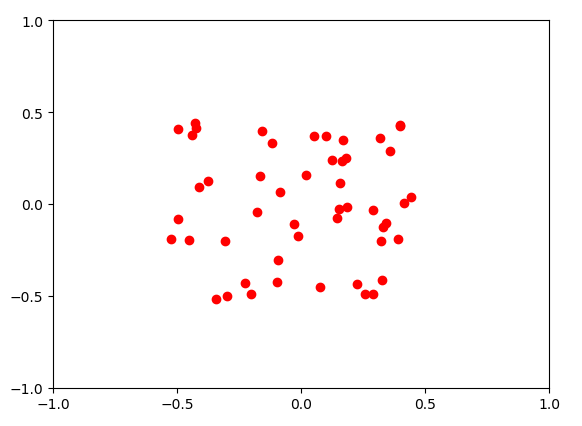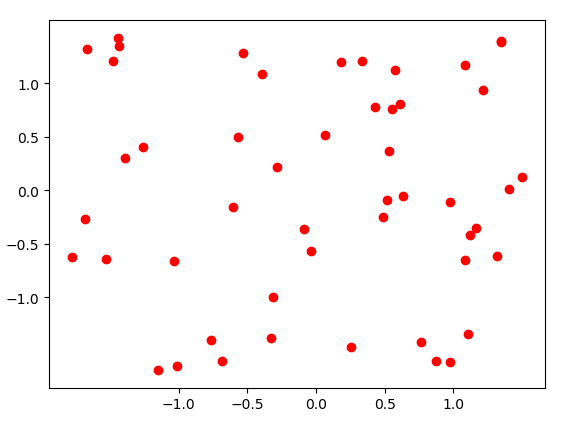2017-03-06 22:29:31 qq_23981335 阅读数 8261
• ###### 深度学习的数据科学和自然语言处理教程

该视频教程探索一些自然语言处理技术的最新进展、深度学习在自然语言处理技术领域的应用、实践和数据资源，以及使用英特尔的Nervanan Platform的企业自然语言处理的应用案例和使用英特尔® AI技术的NLP企业精华案例。

543 人正在学习 去看看 CSDN讲师

1）简单缩放 （Simple Rescaling

2）逐样本均值消减（Per-example mean subtraction

3）特征标准化（Feature Standardization

PCA/ZCAzero-phase component analysis）白化数据归一化后的一个处理步骤就是数据白化。关于白化的基本知识可参考教程：

2019-06-19 19:33:11 gm_Ergou 阅读数 656
• ###### 深度学习的数据科学和自然语言处理教程

该视频教程探索一些自然语言处理技术的最新进展、深度学习在自然语言处理技术领域的应用、实践和数据资源，以及使用英特尔的Nervanan Platform的企业自然语言处理的应用案例和使用英特尔® AI技术的NLP企业精华案例。

543 人正在学习 去看看 CSDN讲师

``````# -*- coding: UTF-8 -*-
import re
import os
import shutil
from PIL import Image

#打开txt文本
f1=open('F:/TF2/new/txt/1/data-clear-new.txt','r')
txt_path="F:/TF2/new/data-pic/txt/"
f15=open(txt_path+'20.txt','w+')
f20=open(txt_path+'21-25.txt','w+')
f25=open(txt_path+'26-30.txt','w+')
f30=open(txt_path+'31-35.txt','w+')
f35=open(txt_path+'36-40.txt','w+')
f40=open(txt_path+'41-45.txt','w+')
f45=open(txt_path+'45.txt','w+')

#获取年龄和文件名
file_split=line.split() #以空格为分割符，把关键词分割出来

old_name=file_split   #原文件名
old_back=os.path.splitext(old_name)  #分离文件名与后缀
# print(old_back)

age=int(file_split)   #年龄
# print(age)

sub=file_split   #性别
# print(sub)

folder=file_split   #原文件夹名称
# print(folder)
root_path="F:/TF2/new/tf2-data-clear/"
save_path = "F:/TF2/new/data-pic/"
if age <= 20:
print(line)
f15.write(line)
shutil.move(root_path + old_name, save_path + "20/" + old_name)

if age >= 21 and age <= 25:
f20.write(line)
shutil.move(root_path + old_name, save_path + "21-25/" + old_name)

if age >= 26 and age <= 30:
f25.write(line)
shutil.move(root_path + old_name, save_path + "26-30/" + old_name)

if age >= 31 and age <= 35:
f30.write(line)
shutil.move(root_path + old_name, save_path + "31-35/" + old_name)

if age >= 36 and age <= 40:
f35.write(line)
shutil.move(root_path + old_name, save_path + "36-40/" + old_name)

if age >= 41 and age <= 45:
f40.write(line)
shutil.move(root_path + old_name, save_path + "41-45/" + old_name)

if age > 45:
f45.write(line)
shutil.move(root_path + old_name, save_path + "45/" + old_name)

f1.close()
f20.close()
f25.close()
f30.close()
f35.close()
f40.close()
f45.close()
``````

2019-01-08 11:18:10 qq_23149979 阅读数 378
• ###### 深度学习的数据科学和自然语言处理教程

该视频教程探索一些自然语言处理技术的最新进展、深度学习在自然语言处理技术领域的应用、实践和数据资源，以及使用英特尔的Nervanan Platform的企业自然语言处理的应用案例和使用英特尔® AI技术的NLP企业精华案例。

543 人正在学习 去看看 CSDN讲师

# 1 创建

## 1.1 单个csv数据集文件创建

``````import os
import numpy as np
import cv2
import tensorflow as tf

folderpath='./data/'
folddirs = os.listdir(folderpath)
all_train_count=0
all_test_count=0
all_count=0
trainrate=0.9
interval=int(10*trainrate+1)
x_train = []
x_test = []
y_train = []
y_test = []

def Image2Vector(img):
temp = cv2.split(img)
temp = np.array(temp, dtype='float') / 255.0
img_vector = temp.reshape(temp.size, order='F')
img_vector = np.transpose([img_vector])
return img_vector
def Label2Vector(label):
y=np.zeros(10)
for i in range(10):
if i==int(label):
y[i]=1.0
else:
y[i]=0.0
return y

for picfoldername in folddirs:
picfilepath=folderpath+picfoldername
picfiledirs = os.listdir(picfilepath)
train_num = int(len(picfiledirs) * trainrate)
train_count = 0
subtotal=0
test_num = len(picfiledirs) - train_num
test_count=0
label=int(picfoldername)
for picfilename in picfiledirs:
img_path = picfilepath + '/' + picfilename
img_vector=Image2Vector(img)
lab_vector=Label2Vector(label)
all_count = all_count + 1
if subtotal%interval!=0:
x_train.append(img_vector)
y_train.append(lab_vector)
all_train_count=all_train_count+1
train_count=train_count+1
else:
x_test.append(img_vector)
y_test.append(lab_vector)
all_test_count = all_test_count + 1
test_count = test_count + 1
subtotal = subtotal + 1
x1train=np.array(x_train)
xtrain=np.zeros([x1train.shape,x1train.shape])
for i in range(x1train.shape):
for j in range(x1train.shape):
xtrain[i,j]=x1train[i,j]
ytrain=np.array(y_train)
x1test=np.array(x_test)
xtest=np.zeros([x1test.shape,x1test.shape])
for i in range(x1test.shape):
for j in range(x1test.shape):
xtest[i,j]=x1test[i,j]
ytest=np.array(y_test)
np.savetxt('./temp/xtrain.csv', xtrain, delimiter=',')
np.savetxt('./temp/ytrain.csv', ytrain, delimiter=',')
np.savetxt('./temp/xtest.csv', xtest, delimiter=',')
np.savetxt('./temp/ytest.csv', ytest, delimiter=',')

print(all_count,all_train_count,all_test_count)

``````

## 1.2 多个csv数据集文件创建

``````import os
import numpy as np
import cv2
import tensorflow as tf

folderpath='./data/'
folddirs = os.listdir(folderpath)
all_train_count=0
all_test_count=0
all_count=0

all_batch_num = 10  #生成10套csv数据

def Image2Vector(img):
temp = cv2.split(img)
temp = np.array(temp, dtype='float') / 255.0
img_vector = temp.reshape(temp.size, order='F')
img_vector = np.transpose([img_vector])
return img_vector
def Label2Vector(label):
y=np.zeros(10)
for i in range(10):
if i==int(label):
y[i]=1.0
else:
y[i]=0.0
return y

for batch_num in range(all_batch_num):

print ("循环第 %d 次" % batch_num)
x_train = []
x_test = []
y_train = []
y_test = []
for picfoldername in folddirs:
picfilepath=folderpath+picfoldername
picfiledirs = os.listdir(picfilepath)

label=int(picfoldername)

file_num = 0
for picfilename in picfiledirs:
img_path = picfilepath + '/' + picfilename
img_vector=Image2Vector(img)
lab_vector=Label2Vector(label)
all_count = all_count + 1

if file_num % 11 == 0:
x_test.append(img_vector)
y_test.append(lab_vector)
all_test_count = all_test_count + 1

elif file_num % 11 == batch_num + 1:
x_train.append(img_vector)
y_train.append(lab_vector)
all_train_count = all_train_count + 1

file_num += 1

x1train = np.array(x_train)

xtrain = np.zeros([x1train.shape,x1train.shape])
for i in range(x1train.shape):
for j in range(x1train.shape):
xtrain[i,j]=x1train[i,j]
ytrain = np.array(y_train)
x1test = np.array(x_test)
xtest = np.zeros([x1test.shape,x1test.shape])
for i in range(x1test.shape):
for j in range(x1test.shape):
xtest[i,j]=x1test[i,j]
ytest=np.array(y_test)

np.savetxt('./temp/xtrain_'+'%d.csv' % batch_num, xtrain, delimiter=',')
np.savetxt('./temp/ytrain_'+'%d.csv' % batch_num, ytrain, delimiter=',')

print ("+++++++++ 第 %d 组训练集文件生成+++++++++"%batch_num)
if batch_num == 9:
np.savetxt('./temp/xtest.csv', xtest, delimiter=',')
np.savetxt('./temp/ytest.csv', ytest, delimiter=',')
print ("+++++++++ 第 %d 组测试集文件生成+++++++++" % batch_num)
print(all_count,all_train_count,all_test_count)
``````

# 3 声明

2019-01-12 20:51:39 qq_35008279 阅读数 794
• ###### 深度学习的数据科学和自然语言处理教程

该视频教程探索一些自然语言处理技术的最新进展、深度学习在自然语言处理技术领域的应用、实践和数据资源，以及使用英特尔的Nervanan Platform的企业自然语言处理的应用案例和使用英特尔® AI技术的NLP企业精华案例。

543 人正在学习 去看看 CSDN讲师

#### 1. 单词和字符的one-hot编码

##### 1）单词级
``````import numpy as np

samples = ['The cat sat on the mat.', 'The dog ate my homework.']  # 初始数据，本例中是一个句子，当然也可以是一篇文章

token_index = {}   # 构建数据中所有标记的索引
for sample in samples:
for word in sample.split():   # 用split方法对样本进行分词，实际应用中，可能还需要考虑到标点符号
if word not in token_index:

token_index[word] = len(token_index) + 1  #为每个唯一单词指定唯一索引，注意我们没有为索引编号0指定单词

max_length = 10   # 对样本进行分词，只考虑样本前max_length单词

results = np.zeros((len(samples), max_length, max(token_index.values()) + 1))    # 将结果保存到results中
for i, sample in enumerate(samples):
for j, word in list(enumerate(sample.split()))[:max_length]:
index = token_index.get(word)
results[i, j, index] = 1.
``````
##### 2）字符集
``````import string

samples = ['The cat sat on the mat.', 'The dog ate my homework.']
characters = string.printable   # 所有可打印的ASCII字符
token_index = dict(zip(characters, range(1, len(characters) + 1)))

max_length = 50
results = np.zeros((len(samples), max_length, max(token_index.values()) + 1))
for i, sample in enumerate(samples):
for j, character in enumerate(sample[:max_length]):
index = token_index.get(character)
results[i, j, index] = 1.
``````
##### 3）Keras实现
``````from keras.preprocessing.text import Tokenizer

samples = ['The cat sat on the mat.', 'The dog ate my homework.']

tokenizer = Tokenizer(num_words=1000)  # i创建一个分词器（tokenizer），设置为只考虑前1000个最常见的单词

tokenizer.fit_on_texts(samples)   # 构建索引单词

sequences = tokenizer.texts_to_sequences(samples)   # 将字符串转换为整数索引组成的列表

one_hot_results = tokenizer.texts_to_matrix(samples, mode='binary')  #可以直接得到one-hot二进制表示。这个分词器也支持除
# one-hot编码外其他向量化模式

word_index = tokenizer.word_index  # 得到单词索引
print('Found %s unique tokens.' % len(word_index))
``````

#### 2. 使用词嵌入

2019-12-01 10:53:41 qq_41084756 阅读数 160
• ###### 深度学习的数据科学和自然语言处理教程

该视频教程探索一些自然语言处理技术的最新进展、深度学习在自然语言处理技术领域的应用、实践和数据资源，以及使用英特尔的Nervanan Platform的企业自然语言处理的应用案例和使用英特尔® AI技术的NLP企业精华案例。

543 人正在学习 去看看 CSDN讲师

# 一、中心化/零均值化

``````import numpy as np
import matplotlib.pyplot as plt
from numpy import random

x = random.rand(50,2)     #随机生成一个50*2（50个样本，2维）的实数矩阵
# print(x)
plt.scatter(x[:,1],x[:,0],color="red")  #以x的第二列为各点的横坐标，第一列为纵坐标
new_ticks = np.linspace(-1, 1, 5)		#固定坐标轴在-1到1之间，共5个值
plt.xticks(new_ticks)
plt.yticks(new_ticks)
plt.show()

#***************零值化******************#
x -= np.mean(x,axis = 0)  #去均值
#print(x)
plt.scatter(x[:,1],x[:,0],color="black")
new_ticks = np.linspace(-1, 1, 5)
plt.xticks(new_ticks)
plt.yticks(new_ticks)
plt.show()
``````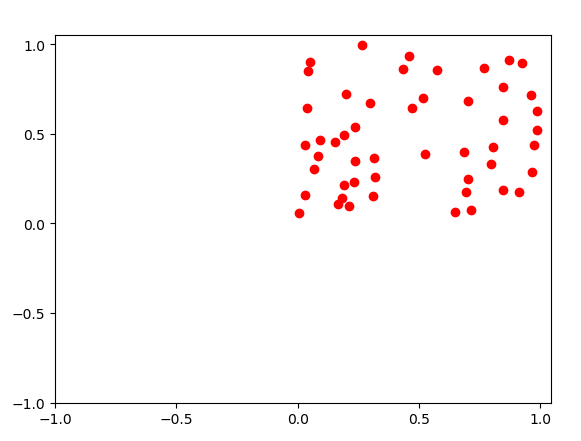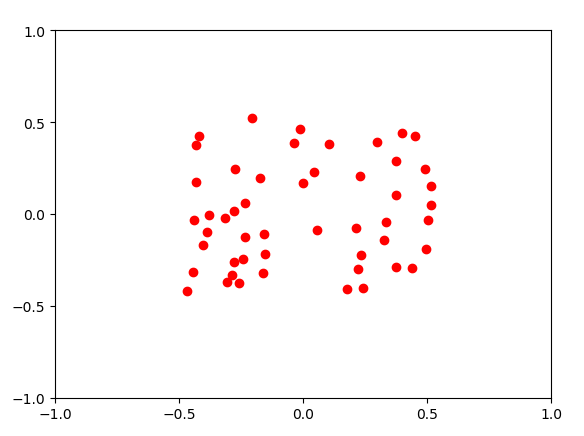# 二、归一化

• 原始数据除以数据绝对值的最大值，以保证所有的数据归一化后都在-1到1之间。
• 原始数据零均值后，再将每一维的数据除以每一维数据的标准差。
这里利用python实现第二种：
``````import numpy as np
import matplotlib.pyplot as plt
from numpy import random

x = random.rand(50,2)
# print(x)
plt.scatter(x[:,1],x[:,0],color="red")
new_ticks = np.linspace(-1, 1, 5)
plt.xticks(new_ticks)
plt.xticks(new_ticks)
plt.show()

#***************归一化******************#
x -= np.mean(x,axis = 0)  #去均值
x /= np.std(x)   #归一化
#print(x)
plt.scatter(x[:,1],x[:,0],color="red")
new_ticks = np.linspace(-1, 1, 5)
plt.xticks(new_ticks)
plt.yticks(new_ticks)
plt.show()
``````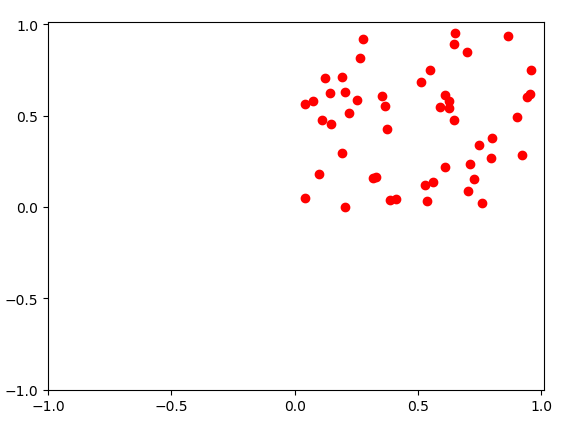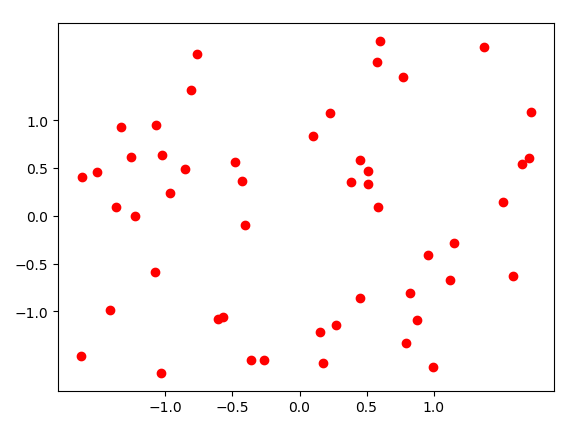# 三、PCA和白化

PCA：主成成分分析，首先将数据变成0均值的，然后计算数据的协方差矩阵来得到数据不同维度之间的相关性，协方差矩阵的第(i,j)个元素表示数据第i维和第j维特征的相关性，特别地，对角线上的元素表示方差。另外，协方差矩阵是对称并且半正定的。可以对该协方差矩阵进行SVD分解，其中U矩阵的列为特征向量，S对角线上的元素是奇异值（等同于特征值的平方）。为了对数据去相关，首先将数据（0均值后的）投影到特征向量上，注意，U的列是相互正交的向量，也因此它们可以被看成基向量。这种投影相当于将数据X旋转、投影到新的基向量轴上。如果再去计算Xrot的协方差矩阵的话，就会发现它是一个对角阵，说明不同维度之间不再相关。np.linalg.svd的一个很好的特性在于返回的U是按照其特征值的大小排序的，排在前面的就是主方向，因此可以通过选取前几个特征向量来减少数据的维度。

``````import numpy as np
import matplotlib.pyplot as plt
from numpy import random

x = random.rand(50,2)
# print(x)
plt.scatter(x[:,1],x[:,0],color="red")
new_ticks = np.linspace(-1, 1, 5)
plt.xticks(new_ticks)
plt.yticks(new_ticks)
plt.show()

#***************PCA和白化***************#
x -= np.mean(x) # 去均值
cov = np.dot(x.T, x) / x.shape   # 计算协方差矩阵得到相关性
U,S,V = np.linalg.svd(cov)			# 协方差矩阵奇异值分解
Xrot = np.dot(x, U)					# 去相关
Xrot_reduced = np.dot(x,U[:,:100])	# 降维
plt.scatter(Xrot_reduced[:,1],Xrot_reduced[:,0], color = "green")
new_ticks = np.linspace(-1, 1, 5)
plt.xticks(new_ticks)
plt.yticks(new_ticks)
plt.show()
#白化数据
Xwhite = Xrot / np.sqrt(S + 1e-5)
plt.scatter(Xwhite[:,1],Xwhite[:,0], color = "purple")
new_ticks = np.linspace(-1, 1, 5)
plt.xticks(new_ticks)
plt.yticks(new_ticks)
plt.show()
``````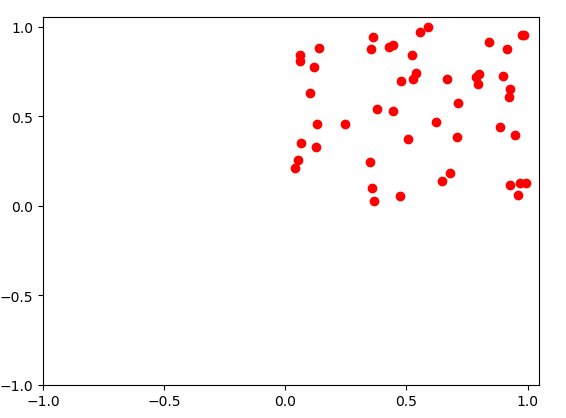PCA处理后：